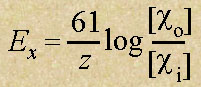Membrane Potential (Contd....)

 If the membrane was solely permeable to potassium the magnitude of the potential difference would be -90mV. The negative sign infront of the potential difference, indicates that the intracellular space is negative in respect to the extracellular space. Another way of looking at this difference is that an electrical potential that is exactly equal but of opposite charge to the membrane potential would block the diffusion of the particular ion involved. Since the magnitude of the diffusion depends on ratio of the concnetrations between the extracellular and intracellular space, then an equation can be built to calculate this potential. This is called the Nernst Equation. A simplified equation at room temperature:where Ex = equilibrium potential in millivolts (mV) for ion x co = concentration of the ion outside the cell ci = concentration of the ion outside the cell z = Valence of the ion (+1 for Na+ or K+) Using the Nernst Equation the equilibrium potential for a cation is negative when the internal concentration is higher than the external concnetration. Thus if the membrane is fully permeable to potassium, then the membrane potential would be negative whilst if the membrane was solely permeable to sodium, then the potential would be positive. Thus in the case of the cellular resting membrane potential, the Potassium Equilibirum Potential is -90mV. If the membrane was fully permeable to Sodium, then the Sodium Equilibrium Potential would be +60mV.